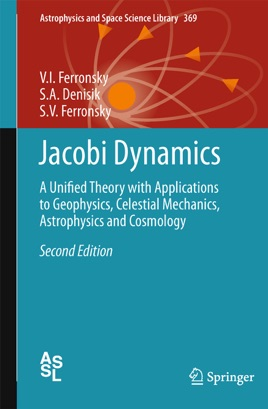• \$169.99

## Publisher Description

In their approach to Earth dynamics the authors consider the fundamentals of Jacobi Dynamics (1987, Reidel) for two reasons. First, because satellite observations have proved that the Earth does not stay in hydrostatic equilibrium, which is the physical basis of today’s treatment of geodynamics. And secondly, because satellite data have revealed a relationship between gravitational moments and the potential of the Earth’s outer force field (potential energy), which is the basis of Jacobi Dynamics. This has also enabled the authors to come back to the derivation of the classical virial theorem and, after introducing the volumetric forces and moments, to obtain a generalized virial theorem in the form of Jacobi’s equation. Thus a physical explanation and rigorous solution was found for the famous Jacobi’s equation, where the measure of the matter interaction is the energy.
The main dynamical effects which become understandable by that solution can be summarized as follows:
the kinetic energy of oscillation of the interacting particles which explains the physical meaning and nature of the gravitation forces;
separation of the shell’s rotation of a self-gravitating body with respect to the mass density;
difference in angular velocities of the shell rotation;
continuity in changing the potential of the outer gravitational force field together with changes in density distribution of the interacting masses (volumetric center of masses);
the nature of the precession of the Earth, the Moon and satellites; the nature of the rotating body’s magnetic field and the generation of the planet’s electromagnetic field.
As a final result, the creation of the bodies in the Solar System having different orbits was discussed. This result is based on the discovery that all the averaged orbital velocities of the bodies in the Solar System and the Sun itself are equal to the first cosmic velocities of their proto-parents during the evolution of their redistributed mass density.

Audience
The work is a logical continuation of the book Jacobi Dynamics and is intended for researchers, teachers and students engaged in theoretical and experimental research in various branches of astronomy (astrophysics, celestial mechanics and stellar dynamics and radiophysics), geophysics (physics and dynamics of the Earth’s body, atmosphere and oceans), planetology and cosmogony, and for students of celestial, statistical, quantum and relativistic mechanics and hydrodynamics.